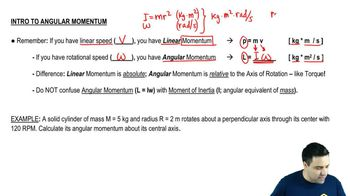Start typing, then use the up and down arrows to select an option from the list.
3:29 minutes
Problem 10c
Textbook Question

# CALC A hollow, thin-walled sphere of mass 12.0 kg and diameter 48.0 cm is rotating about an axle through its center. The angle (in radians) through which it turns as a function of time (in seconds) is given by θ(t) = At^2 + Bt^4, where A has numerical value 1.50 and B has numerical value 1.10. (a) What are the units of the constants A and B?Verified Solution
This video solution was recommended by our tutors as helpful for the problem above.
97views